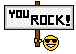• Get access

# Requests & Ideas - page 402193

Thank you Maestro. Well done

Is it possible to see the velocity separate, not mixed with the initial line?

rosalieone

This is when the velocity of the indicator is added to the indicator itself : trend_analysis_-_historical_-_smoothed_2.1.mq4

____________________

Summing the "velocity + acceleration" to the indicator value would be doubling the already existing momentum that is used in the indicator )as I told - that "velocity" is simply a momentum, the guy has mixed up some thing when he told that it has not been done yet)163386

rosalieone:
Thank you Maestro. Well done Is it possible to see the velocity separate, not mixed with the initial line?

Let me find out some visual mode that will not interfere with the main indicator value193

Nothing special, just in a new window under if possible, thanks

Let me find out some visual mode that will not interfere with the main indicator value163386

rosalieone:
Nothing special, just in a new window under if possible, thanks

This is one way it can be done : trend_analysis_-_historical_-_smoothed_2.2.mq4

Files:193

Thank you perfect !!!193

rosalieone:
Thank you perfect !!!Dear Mladen, i'm testing the indi.

Question, should the speed line not cross the zero line when the upper indicator start reversing ?163386

rosalieone:
Dear Mladen, i'm testing the indi. Question, should the speed line not cross the zero line when the upper indicator start reversing ?

rosalieone

If I want to keep both values visible, then speed line had to be moved bellow zero. Add level -0.5 and try to use that levels for that type of crosses193

rosalieone If I want to keep both values visible, then speed line had to be moved bellow zero. Add level -0.5 and try to use that levels for that type of crosses

Yes, i understand that in order to see both lines you managed to tweak it wisely that way. What i was meaning is that the speed should not move in paralel with the upper indicator, in fact as i understand how speed is calculated it should cross the zero line when the upper indicator reverse. (as acceleration cross the zero line when speed reverse). What do you think ?163386

rosalieone:
Yes, i understand that in order to see both lines you managed to tweak it wisely that way. What i was meaning is that the speed should not move in paralel with the upper indicator, in fact as i understand how speed is calculated it should cross the zero line when the upper indicator reverse. (as acceleration cross the zero line when speed reverse). What do you think ?

rosalieone

That indicator is not calculating the spread, it is calculating the difference between a value and value n bars ago (that is why I told that it is a simple momentum). When the formula for "velocity" indicator is applied to the values of the trend analysis historical indicator, those are the values calculated193